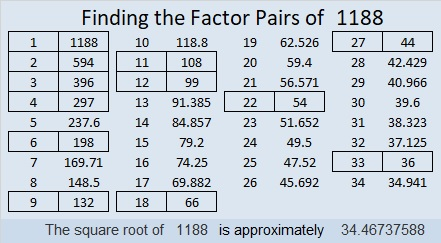# 1188 How Many Triangles? How Many Factors?

Manually counting ALL the triangles in the graphic below could get confusing. Try counting all the triangles that point up first then all the triangles that point down.Since the number of triangle rows is an even number, you could just use this formula:

n(n+2)(2n+1)/8 = the number of triangles

In this case, n = 16, so the number of triangles is 16×18×33/8 = 1188.

(If the number of rows is an odd number, the formula produces a number with a decimal. In that case, just ignore the decimal and everything after it to get the number of triangles.)

• 1188 is a composite number.
• Prime factorization: 1188 = 2 × 2 × 3 × 3 × 3 × 11, which can be written 1188 = 2² × 3³ × 11
• The exponents in the prime factorization are 2, 3 and 1. Adding one to each and multiplying we get (2 + 1)(3 + 1)(1 + 1) = 3 × 4 × 2 = 24. Therefore 1188 has exactly 24 factors.
• Factors of 1188: 1, 2, 3, 4, 6, 9, 11, 12, 18, 22, 27, 33, 36, 44, 54, 66, 99, 108, 132, 198, 297, 396, 594, 1188
• Factor pairs: 1188 = 1 × 1188, 2 × 594, 3 × 396, 4 × 297, 6 × 198, 9 × 132, 11 × 108, 12 × 99, 18 × 66, 22 × 54, 27 × 44 or 33 × 36
• Taking the factor pair with the largest square number factor, we get √1188 = (√36)(√33) = 6√33 ≈ 34.467381188 has MANY possible factor trees. Here are four of them:1188 is an interesting-looking base 10 number, but it also looks interesting when it is written in some other bases:
It’s 2244 in BASE 8,
990 in BASE 11 because 9(11²) + 9(11) = 9(132) = 1188,
543 in BASE 15,
4A4 in BASE 16 (A is 10 base 10),
XX in BASE 35 (X is 33 base 10) because 33(35) + 33(1) = 33(36) = 1188,
and it’s X0 in BASE 36 because 33(36) = 1188

This site uses Akismet to reduce spam. Learn how your comment data is processed.